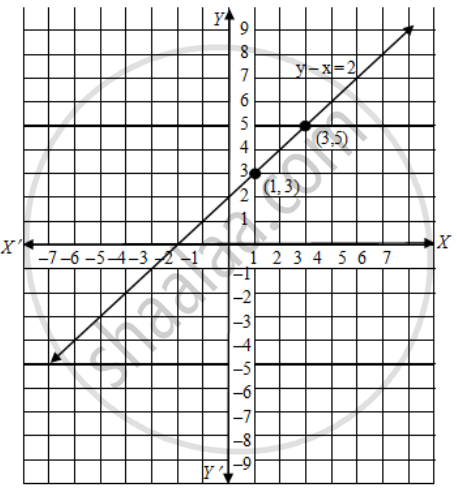Share

# Draw the graph of the equation y – x = 2 - CBSE Class 10 - Mathematics

ConceptPair of Linear Equations in Two Variables

#### Question

Draw the graph of the equation y – x = 2

#### Solution

We have,

y – x = 2

⇒ y = x + 2

When x = 1, y = 1 + 2 = 3

When x = 3, y = 3 + 2 = 5

Thus, we have the following table exhibiting the abscissa and ordinates of points on the line represented by the given equation. Plotting the points (1, 3) and (3, 5) on the graph paper and drawing a line joining them, we obtain the graph of the line represented by the given equation as shown in Fig.Is there an error in this question or solution?

#### Video TutorialsVIEW ALL 

Solution Draw the graph of the equation y – x = 2 Concept: Pair of Linear Equations in Two Variables.
S